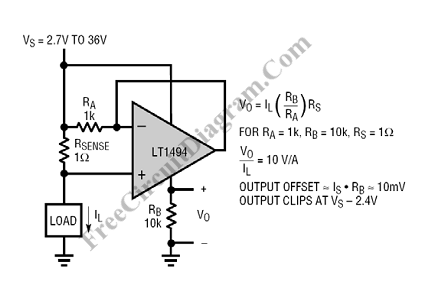# Self-Powered Current Sensing CircuitThis current sensing circuit sense the current at the high side (between positive supply and load). This circuit take the benefit of the microampere supply current and Rail-to-Rail input of the LT1494 op-amp. Here is the schematic diagram of the circuit:Look at the op-amp, the inverting input is connected directly to the output, and this negative feedback will keep the inverting input to be in the same level with the noninverting input. This mans that the voltage across RA will be kept equal to the voltage across Rsense.  This voltage make the current flows from the power source source to the output pin of the op-amp through RA, and finally return to ground via RB. Almost all of the current at RB comes from RA since the internal circuit draws only very small amount of current through Vcc pin, so the output at RB is valid. [Circuit schematic diagram source: Linear Technology Application Notes]